# Triangle - math word problems

1. Rotary coneThe volume of the rotation of the cone is 472 cm3 and angle between the side of the cone and base angle is 70°. Calculate lateral surface area of this cone.
2. Diagonals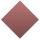Rhombus has two diagonals e=14 dm and f=11 dm. Calculate the side angle and height of the rhombus.
3. Oil rigOil drilling rig is 23 meters height and fix the ropes which ends are 7 meters away from the foot of the tower. How long are these ropes?
4. Boat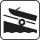A force of 300 kg (3000 N) is required to pull a boat up a ramp inclined at 14° with horizontal. How much does the boat weight?
5. MapleMaple peak is visible from a distance 3 m from the trunk from a height of 1.8 m at angle 62°. Determine the height of the maple.
6. CanopyMr Peter has metal roof cone shape with a height of 127 cm and radius 130 cm over well. He needs paint the roof with anticorrosion. How many kg of color must he buy if the manufacturer specifies the consumption of 1 kg to 3.3 m2?
7. n-gon IIWhat is the side length of the regular 5-gon circumscribed circle of radius 11 cm?
8. n-gonWhat is the side length of the regular 5-gon inscribed in a circle of radius 12 cm?
9. Block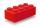Calculate the volume of a cuboid ABCDEFGH if |AB| = 16 cm, |BC| = 19 cm and the angle ∠CDG = 36.9°
10. ConeCalculate volume and surface area of ​​the cone with a diameter of the base d = 15 cm and side of cone with the base has angle 52°.
11. TowerHow many m2 of copper plate should be to replace roof of the tower conical shape with diameter 24 m and the angle at the vertex of the axial section is 144°?
12. DiagonalCalculate the length of the diagonal of the rectangle ABCD with sides a = 8 cm, b = 7 cm.
13. ArmCalculate the length of the arm r of isosceles triangle ABC, with base |AB| = 14 cm and a height v=18 cm.
14. ThalesCalculate the length of the Thales' circle described to right triangle with hypotenuse 18.4 cm.
15. Gon functions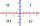Decide which of the numbers (values ​​of trigonometric functions) are positive and which are negative (or zero). Positive mark +1 and negative -1.
16. BaseCompute base of an isosceles triangle, with the arm a=20 cm and a height above the base h=10 cm.
17. TetrahedronCalculate height and volume of a regular tetrahedron whose edge has a length 4 cm.
18. IS trapezoid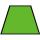Isosceles trapezoid arm measured 35 cm. Height is 30 cm and middle segment is 65 cm. Determine length of its bases.Road embankment has a cross section shape of an isosceles trapezoid with bases 5 m and 7 m, and 2 m long leg. How many cubic meters of soil is in embankment length of 1474 meters?
20. Area of RT 2Calculate the area of right triangle whose legs have a length 5.8 cm and 5.8 cm.

Do you have an interesting mathematical word problem that you can't solve it? Submit math problem, and we can try to solve it.

We will send a solution to your e-mail address. Solved examples are also published here. Please enter the e-mail correctly and check whether you don't have a full mailbox.

Please do not submit problems from current active competitions such as Mathematical Olympiad, correspondence seminars etc...